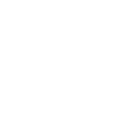×6th Unit

### 12th Class Mathematics Unit 6 Test

Here you can prepare 12th Class Mathematics Unit 6 Conic Section Test. Click the button for 100% free full practice test.

## Second Year Mathematics Chapter 6 Online MCQ Test for 2nd Year Mathematics Unit 6 Conic Section Preparation

This online test contains MCQs about following topics:.

Introduction - Tangents and Normals - Analytical Proofs of Important Properties of a Circle - Parabola - Ellipse and Its Elements - Hyperbola and Its Elements - Tangents and Normals - Translation and Rotation of Axes - The General Equation of Second Degree

FSC Part 2 Mathematics Unit 6 Test### Second Year Mathematics Chapter 6 Online MCQ Test for 2nd Year Mathematics Unit 6 Conic Section Preparation

1
• A.
• B.
• C.
• D.
2 The axis of the parabola y<sup>2</sup> = 4ax is:
• A. x = 0
• B. x = a
• C. y = 0
• D. y = a
3 One of the angles of a triangle inscribed in a circle is of 40°. If one of its' the diameter, the other angles have the measures:
• A. 30°, 110°
• B. 40°, 100°
• C. 50°, 90°
• D. 20°, 120°
4 In the case of translation of axes which formula is true:
• A. x = X - h
• B. x = X + h
• C. x + X = h
• D. None
5 If the equation of the parabola is y<sup>2</sup> = - 4ax, then opening of the parabola is to the ______ of the y-axis:
• A. Left
• B. Upward
• C. Right
• D. Downward
6 A line perpendicular to a radial chord of a circle at the end-point (which lies on the circle) is a:
• A. Secant
• B. Diameter
• C. Chord
• D. Tangent
7 Measure of the central angle of a minor arc is ____ the measure of the angle subtended in the corresponding major arc.
• A. Equal
• B. Double
• C. Not equal to
• D. Triple
8 If r is the radius of any circle and C its center, then any point P(x<sub>1</sub>, y<sub>1</sub>) lies outside the circle only if:
• A. |CP| &lt; r
• B. |CP| = r
• C. |CP| &gt; r
• D. None of these
9
• A. x = 0
• B. y = -a
• C. y = 0
• D. y = -a
10 The center of circle x<sup>2</sup> + y<sup>2</sup> + 2gx + 2fy + c = 0 is:
• A. (-g, -f)
• B. (-f, -g)
• C. (0, 0)
• D. (g, f)

### Top Scorers of Mathematics Fsc Part 2 Chapter 6 Online Test

K

#### Kewal Kumar

Lahore29 - Sep - 2023

20/20
27 Sec
K

#### Kewal Kumar

Lahore29 - Sep - 2023

19/20
27 Sec
K

#### Kewal Kumar

Lahore29 - Sep - 2023

18/20
27 Sec
K

#### Kewal Kumar

Lahore29 - Sep - 2023

17/20
28 Sec
B

#### BILALHSN1

Lahore22 - Nov - 2023

17/20
03 Mins 11 Sec
K

#### Kewal Kumar

Lahore29 - Sep - 2023

16/20
28 Sec
K

#### Kewal Kumar

Lahore29 - Sep - 2023

14/20
28 Sec
B

#### BILALHSN1

Lahore22 - Nov - 2023

14/20
04 Mins 31 Sec
B

#### BILALHSN1

Lahore22 - Nov - 2023

14/20
04 Mins 32 Sec
K

#### Kewal Kumar

Lahore29 - Sep - 2023

13/20
28 Sec
B

#### Bilal Ahmed

Lahore25 - Nov - 2023

13/20
08 Mins 31 Sec
K

#### Kewal Kumar

Lahore29 - Sep - 2023

12/20
28 Sec
K

#### Kewal Kumar

Lahore29 - Sep - 2023

12/20
28 Sec
B

#### BILALHSN1

Lahore22 - Nov - 2023

12/20
04 Mins 54 Sec
K

#### Kewal Kumar

Lahore29 - Sep - 2023

11/20
28 Sec

Sort By:
• T

23 Mar 2019

Student

Like (2)
• W

02 Mar 2019

tes

Like (1)
• N

#### Noman

28 Feb 2016

q

Like (1)
X

to continue to ilmkidunya.comFill the form. Our admission consultants will call you with admission options.

X

to continue to ilmkidunya.com

X

to continue to ilmkidunya.com

X